# powerbw

Power bandwidth

## Syntax

``bw = powerbw(x)``
``bw = powerbw(x,fs)``
``bw = powerbw(pxx,f)``
``bw = powerbw(sxx,f,rbw)``
``bw = powerbw(___,freqrange,r)``
``````[bw,flo,fhi,power] = powerbw(___)``````
``powerbw(___)``

## Description

````bw = powerbw(x)` returns the 3-dB (half-power) bandwidth, `bw`, of the input signal, `x`.```

example

````bw = powerbw(x,fs)` returns the 3-dB bandwidth in terms of the sample rate, `fs`.```

example

````bw = powerbw(pxx,f)` returns the 3-dB bandwidth of the power spectral density (PSD) estimate, `pxx`. The frequencies, `f`, correspond to the estimates in `pxx`.```
````bw = powerbw(sxx,f,rbw)` computes the 3-dB bandwidth of the power spectrum estimate, `sxx`. The frequencies, `f`, correspond to the estimates in `sxx`. `rbw` is the resolution bandwidth used to integrate each power estimate.```
````bw = powerbw(___,freqrange,r)` specifies the frequency interval over which to compute the reference level. This syntax can include any combination of input arguments from previous syntaxes, as long as the second input argument is either `fs` or `f`. If the second input is passed as empty, normalized frequency will be assumed. `freqrange` must lie within the target band.If you also specify `r`, the function computes the difference in frequency between the points where the spectrum drops below the reference level by `r` dB or reaches an endpoint.```

example

``````[bw,flo,fhi,power] = powerbw(___)``` also returns the lower and upper bounds of the power bandwidth and the power within those bounds.```
````powerbw(___)` with no output arguments plots the PSD or power spectrum in the current figure window and annotates the bandwidth.```

## Examples

collapse all

Generate 1024 samples of a chirp sampled at 1024 kHz. The chirp has an initial frequency of 50 kHz and reaches 100 kHz at the end of the sampling. Add white Gaussian noise such that the signal-to-noise ratio is 40 dB.

```nSamp = 1024; Fs = 1024e3; SNR = 40; t = (0:nSamp-1)'/Fs; x = chirp(t,50e3,nSamp/Fs,100e3); x = x + randn(size(x))*std(x)/db2mag(SNR);```

Estimate the 3-dB bandwidth of the signal and annotate it on a plot of the power spectral density (PSD).

`powerbw(x,Fs)`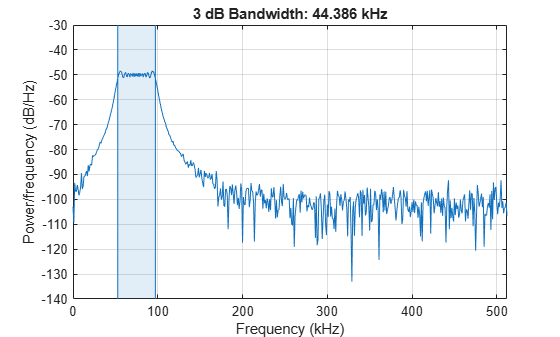```ans = 4.4386e+04 ```

Generate another chirp. Specify an initial frequency of 200 kHz, a final frequency of 300 kHz, and an amplitude that is twice that of the first signal. Add white Gaussian noise.

```x2 = 2*chirp(t,200e3,nSamp/Fs,300e3); x2 = x2 + randn(size(x2))*std(x2)/db2mag(SNR);```

Concatenate the chirps to produce a two-channel signal. Estimate the 3-dB bandwidth of each channel.

`y = powerbw([x x2],Fs)`
```y = 1×2 104 × 4.4386 9.2208 ```

Annotate the 3-dB bandwidths of the two channels on a plot of the PSDs.

`powerbw([x x2],Fs);`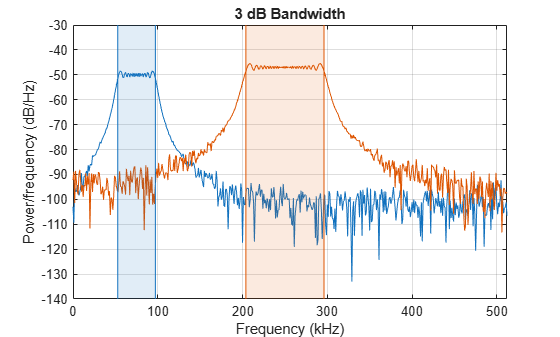Add the two channels to form a new signal. Plot the PSD and annotate the 3-dB bandwidth.

`powerbw(x+x2,Fs)`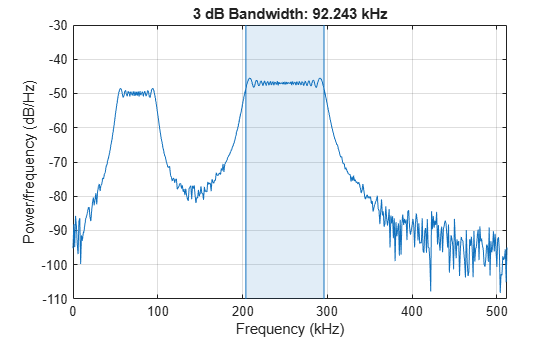```ans = 9.2243e+04 ```

Generate 1024 samples of a 100.123 kHz sinusoid sampled at 1024 kHz. Add white Gaussian noise such that the signal-to-noise ratio is 40 dB. Reset the random number generator for reproducible results.

```nSamp = 1024; Fs = 1024e3; SNR = 40; rng default t = (0:nSamp-1)'/Fs; x = sin(2*pi*t*100.123e3); x = x + randn(size(x))*std(x)/db2mag(SNR);```

Use the `periodogram` function to compute the power spectral density (PSD) of the signal. Specify a Kaiser window with the same length as the signal and a shape factor of 38. Estimate the 3-dB bandwidth of the signal and annotate it on a plot of the PSD.

```[Pxx,f] = periodogram(x,kaiser(nSamp,38),[],Fs); powerbw(Pxx,f);```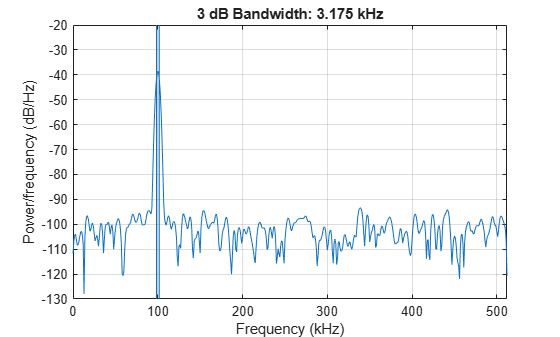Generate another sinusoid, this one with a frequency of 257.321 kHz and an amplitude that is twice that of the first sinusoid. Add white Gaussian noise.

```x2 = 2*sin(2*pi*t*257.321e3); x2 = x2 + randn(size(x2))*std(x2)/db2mag(SNR);```

Concatenate the sinusoids to produce a two-channel signal. Estimate the PSD of each channel and use the result to determine the 3-dB bandwidth.

```[Pyy,f] = periodogram([x x2],kaiser(nSamp,38),[],Fs); y = powerbw(Pyy,f)```
```y = 1×2 103 × 3.1753 3.3015 ```

Annotate the 3-dB bandwidths of the two channels on a plot of the PSDs.

`powerbw(Pyy,f);`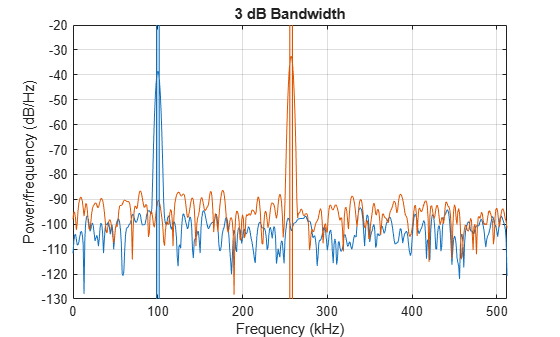Add the two channels to form a new signal. Estimate the PSD and annotate the 3-dB bandwidth.

```[Pzz,f] = periodogram(x+x2,kaiser(nSamp,38),[],Fs); powerbw(Pzz,f);```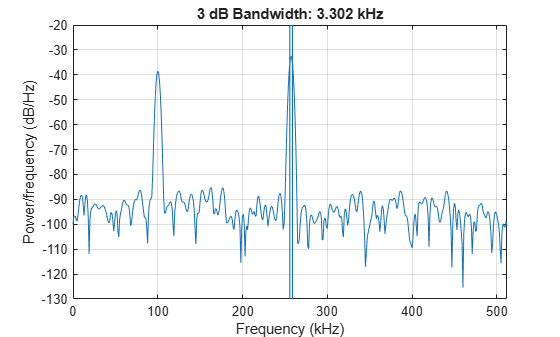Generate a signal whose PSD resembles the frequency response of an 88th-order bandpass FIR filter with normalized cutoff frequencies $0.25\pi$ rad/sample and $0.45\pi$ rad/sample.

`d = fir1(88,[0.25 0.45]);`

Compute the 3-dB occupied bandwidth of the signal. Specify as a reference level the average power in the band between $0.2\pi$ rad/sample and $0.6\pi$ rad/sample. Plot the PSD and annotate the bandwidth.

`powerbw(d,[],[0.2 0.6]*pi,3);`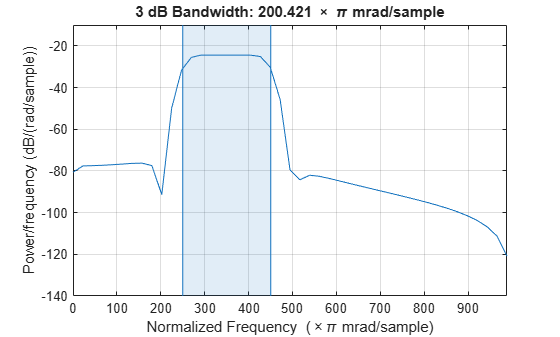Output the bandwidth, its lower and upper bounds, and the band power. Specifying a sample rate of $2\pi$ is equivalent to leaving the rate unset.

```[bw,flo,fhi,power] = powerbw(d,2*pi,[0.2 0.6]*pi); fprintf('bw = %.3f*pi, flo = %.3f*pi, fhi = %.3f*pi \n', ... [bw flo fhi]/pi)```
```bw = 0.200*pi, flo = 0.250*pi, fhi = 0.450*pi ```
`fprintf('power = %.1f%% of total',power/bandpower(d)*100)`
```power = 96.9% of total ```

Add a second channel with normalized cutoff frequencies $0.5\pi$ rad/sample and $0.8\pi$ rad/sample and an amplitude that is one-tenth that of the first channel.

`d = [d;fir1(88,[0.5 0.8])/10]';`

Compute the 6-dB bandwidth of the two-channel signal. Specify as a reference level the maximum power level of the spectrum.

`powerbw(d,[],[],6);`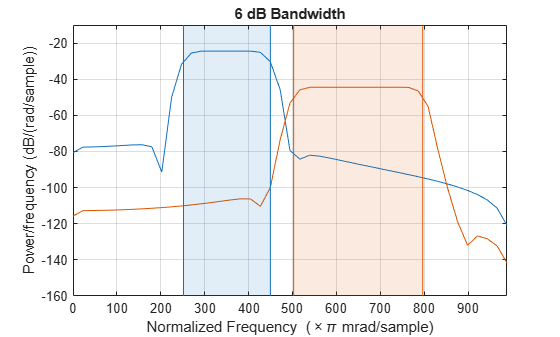Output the 6-dB bandwidth of each channel and the lower and upper bounds.

```[bw,flo,fhi] = powerbw(d,[],[],6); bds = [bw;flo;fhi]; fprintf('One: bw = %.3f*pi, flo = %.3f*pi, fhi = %.3f*pi \n',bds(:,1)/pi)```
```One: bw = 0.198*pi, flo = 0.252*pi, fhi = 0.450*pi ```
`fprintf('Two: bw = %.3f*pi, flo = %.3f*pi, fhi = %.3f*pi \n',bds(:,2)/pi)`
```Two: bw = 0.294*pi, flo = 0.503*pi, fhi = 0.797*pi ```

## Input Arguments

collapse all

Input signal, specified as a vector or matrix. If `x` is a vector, it is treated as a single channel. If `x` is a matrix, then `powerbw` computes the power bandwidth independently for each column. `x` must be finite-valued.

Example: `cos(pi/4*(0:159))+randn(1,160)` is a single-channel row-vector signal.

Example: `cos(pi./[4;2]*(0:159))'+randn(160,2)` is a two-channel signal.

Data Types: `single` | `double`

Sample rate, specified as a positive real scalar. The sample rate is the number of samples per unit time. If the time is measured in seconds, then the sample rate is in hertz.

Data Types: `single` | `double`

Power spectral density (PSD) estimate, specified as a vector or matrix. If `pxx` is a one-sided estimate, then it must correspond to a real signal. If `pxx` is a matrix, then `powerbw` computes the bandwidth of each column of `pxx` independently.

The power spectral density must be expressed in linear units, not decibels. Use `db2pow` to convert decibel values to power values.

Example: ```[pxx,f] = periodogram(cos(pi./[4;2]*(0:159))'+randn(160,2))``` specifies the periodogram PSD estimate of a noisy two-channel sinusoid sampled at 2π Hz and the frequencies at which it is computed.

Data Types: `single` | `double`

Frequencies, specified as a vector. If the first element of `f` is 0, then `powerbw` assumes that the spectrum is a one-sided spectrum of a real signal. In other words, the function doubles the power value in the zero-frequency bin as it seeks the 3-dB point.

Data Types: `single` | `double`

Power spectrum estimate, specified as a vector or matrix. If `sxx` is a matrix, then `obw` computes the bandwidth of each column of `sxx` independently.

The power spectrum must be expressed in linear units, not decibels. Use `db2pow` to convert decibel values to power values.

Example: ```[sxx,w] = periodogram(cos(pi./[4;2]*(0:159))'+randn(160,2),'power')``` specifies the periodogram power spectrum estimate of a two-channel sinusoid embedded in white Gaussian noise and the normalized frequencies at which it is computed.

Data Types: `single` | `double`

Resolution bandwidth, specified as a positive scalar. The resolution bandwidth is the product of two values: the frequency resolution of the discrete Fourier transform and the equivalent noise bandwidth of the window used to compute the PSD.

Data Types: `single` | `double`

Frequency range, specified as a two-element vector of real values. If you specify `freqrange`, then the reference level is the average power level in the reference band. If you do not specify `freqrange`, then the reference level is the maximum power level of the spectrum.

Data Types: `single` | `double`

Power level drop, specified as a positive real scalar expressed in dB.

Data Types: `single` | `double`

## Output Arguments

collapse all

Power bandwidth, returned as a scalar or vector.

• If you specify a sample rate, then `bw` has the same units as `fs`.

• If you do not specify a sample rate, then `bw` has units of rad/sample.

Bandwidth frequency bounds, returned as scalars.

Power stored in bandwidth, returned as a scalar or vector.

## Algorithms

To determine the 3-dB bandwidth, `powerbw` computes a periodogram power spectrum estimate using a rectangular window and takes the maximum of the estimate as a reference level. The bandwidth is the difference in frequency between the points where the spectrum drops at least 3 dB below the reference level. If the signal reaches one of its endpoints before dropping by 3 dB, then `powerbw` uses the endpoint to compute the difference.